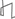Technical Reports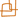Work Products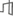Research Abstracts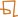Historical Collections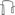Research Abstracts - 2006IntroductionLanguage, Learning, Vision & GraphicsPhysical, Biological & Social SystemsSystemsTheory### On the Similarity of Trees

#### Oren Weimann & Erik Demaine

##### Global Similarity

The problem of comparing trees occurs in diverse areas such as comparisons of structured text databases like XML, computer vision, compiler optimization, natural language processing, and computational biology [3,6,8,9]. For example, the secondary structure of RNA sequences is modeled as ordered labeled trees. Recently, comparing RNA sequences has gained increasing interest due to numerous discoveries of biological functions which are associated with them. A major fraction of the function of an RNA is determined by its secondary structure .

The edit distance metric is a common similarity measure for ordered labeled trees. Let T1 and T2 be two ordered trees, where each vertex is assigned a label from a given alphabet. The edit distance between T1 and T2 is the minimum cost of transforming T1 into T2 by a sequence of elementary operations consisting of deleting and relabeling existing nodes, as well as inserting new nodes. The tree edit distance problem was introduced by Tai  as a generalization of the well known string edit distance problem . Our research goal is to improve on the worst case running time of algorithms that compute tree edit distance<.

Let T1 and T2 be ordered labeled trees such that |T1|=m and |T2|=n, and m < n. We denote by L(Ti) and by D(Ti) the numbers of leaves and the depth of the tree Ti respectively. Tai presented an algorithm that computes the edit distance using O(mnL(T1)^2L(T2)^2) time and space. Shasha and Zhang  improved this result to an O((mn)min(D(T1),L(T1))min(D(T2),L(T2))) time algorithm using O(mn) space. In the worst case, their algorithm runs in O(m^2n^2) time. Klein  improved this result to an O(m^2nlogn) worst case time algorithm that uses O(mn) space. These last two algorithms are based on very similar dynamic programs, and both present different ways of computing only a subset of the DP table; these entries are referred to as relevant subproblems. In , Dulucq and Touzet introduced the notion of a strategy as a general framework for algorithms that use this type of DP, and a lower bound of O(mnlogmlogn) time was proved for any strategy. We are focusing on narrowing the gap between the lower bound and the actual edit distance algorithms by tightening the lower bound and by devising faster algorithms.

##### Local Similarity

Searching for local similarities is at least as important as determining the global similarity in RNA sequences. In contrast, most RNA sequence-structure alignment methods are global. In , we introduce two new local similarity metrics for comparing RNA sequences. We define a local alignment of two RNA sequences which captures both structural and sequential information of an RNA molecule. Furthermore, we present extensions of two well known local alignment metrics used for strings: The Smith Waterman metric , and its normalized version . Following this, we generalize the familiar alignment graph used in string comparison to the case of RNAs, and utilize this generalization to devise two algorithms for computing local similarity according to our suggested metrics. These algorithms run in O(m^2nlogn) and O(m^2nlogn+n^2m)\$ time respectively, and can work with any arbitrary scoring scheme. The m^2nlogn bottleneck of these two algorithms origins in a preprocessing stage that consists of running Klein's algorithm. Thus, improving Klein's global algorithm will also improve the local algorithms.

##### References:

 A.N. Arslan, \"{O}. E\v{g}ecio\u{g}lu, and P.A. Pevzner. "A new approach to sequence alignment: normalized sequence alignment". In Journal of Bioinformatics , 17(4) pp. 327-337, 2001.

 R. Backofen, G. M. Landau, D. Hermelin, and O. Weimann. "Local Alignment of RNA Sequences with Arbitrary Scoring Schemes". In Proceedings of the 17th Annual Symposium on Combinatorial Pattern Matching (CPM), 2006.

 P. Bille. "Tree Edit Distance, Alignment Distance and Inclusion". In Technical report TR-2003-23. IT University of Copenhagen, 2003.

 S. Dulucq and H. Touzet. "Analysis of Tree Edit Distance Algorithms". In The Proceedings of the 14th annual symposium on Combinatorial Pattern Matching (CPM), pp. 83--95, 2003.

 P. N. Klein. "Computing the Edit-Distance between Unrooted Ordered Trees". In The Proceedings of the 6th annual European Symposium on Algorithms (ESA), pp. 91--102, 1998.

 P. N. Klein, S. Tirthapura, D. Sharvit, and B. B. Kimia. "A tree-edit-distance algorithm for comparing simple, closed shapes". In The Proceedings of the 11th ACM-SIAM Symposium on Discrete Algorithms (SODA), pp. 696-704, 2000.

 P.B Moore. "Structural motifs in RNA". In Annual review of biochemistry, 68 pp. 287--300, 1999.

 D. Shasha and K. Zhang. "Simple Fast Algorithms for the Editing Distance Between Trees and Related Problems". In SIAM Journal of Computing, 18(6) pp. 1245--1262, 1989.

 K. Tai. "The Tree-to-Tree Correction Problem". In Journal of the Association for Computing Machinery (JACM), 26(3) pp. 422--433, 1979.

 T.F. Smith and M.S. Waterman. "The identification of common molecular subsequences". In Journal of Molecular Biology, 147 pp. 195--197, 1981.

 R. A. Wagner and M. J. Fischer. "The String-to-String Correction Problem". In Journal of the ACM, 21(1) pp. 168--173, 1974.Computer Science and Artificial Intelligence Laboratory (CSAIL) The Stata Center, Building 32 - 32 Vassar Street - Cambridge, MA 02139 - USA tel:+1-617-253-0073 - publications@csail.mit.edu